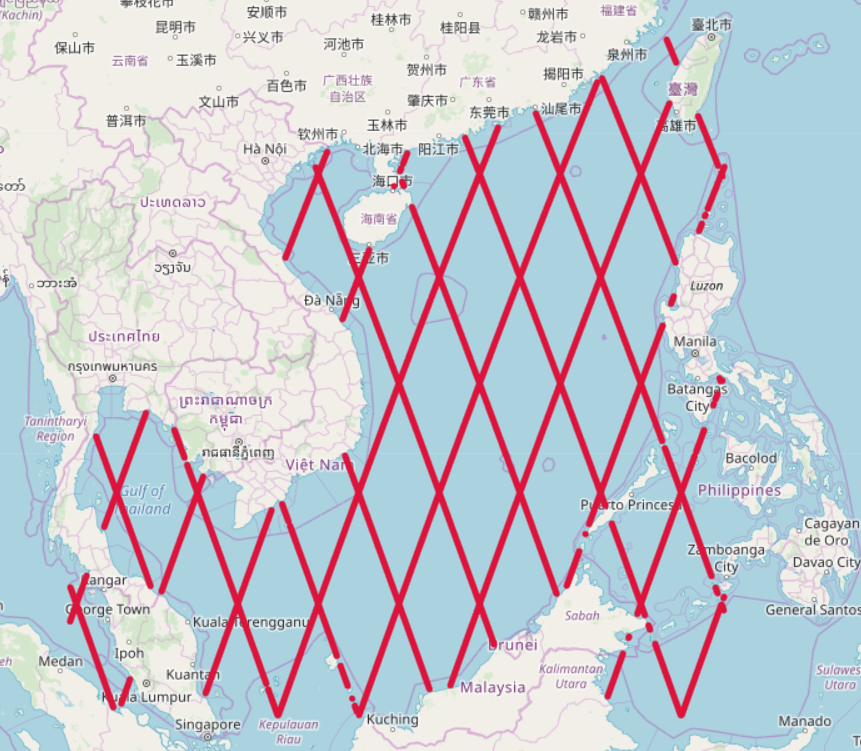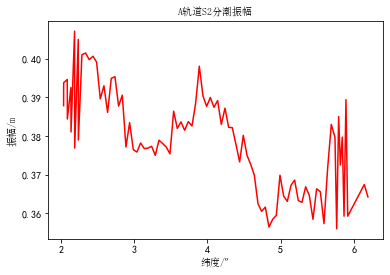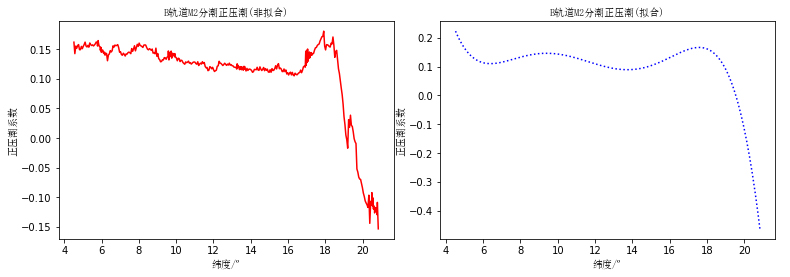## 选题

C题的题干很长，下面仅做部分节选：

1.根据星下观测点各主要分潮（$M_{2},S_{2},K_{1},O_{1}$）的潮汐调和常数，沿轨道作图后，可发现潮汐调和常数在沿轨道方向，在空间有细结构，而此细结构是内潮对正压潮的调制；请设法对沿轨道的各分潮的潮汐调和常数进行正压潮和内潮的分离。

2.利用数据插值或拟合方法给出南海的各主要分潮（$M_{2},S_{2},K_{1},O_{1}$）的同潮图，并利用潮汐验潮点的调和常数给予评价或验证；特别需要指出的是，需明确所利用方法的优势。

1.地形数据，来自ETOPO5，分辨率为$5’ \times 5’$ ，此处数据度范围$2^{\circ} \backsim 25^{\circ} N，99^{\circ} \backsim 122^{\circ} E$

2.中国近海及周边海域217个验潮站的资料，包括4个分潮（$M_{2},S_{2},K_{1},O_{1}$）的潮汐调和常数

3.南海沿轨星下观测点的4个分潮（$M_{2},S_{2},K_{1},O_{1}$）的潮汐调和常数

## 建模

Dushaw(2002)在夏威夷海区采用300km滑动平均的方法进行滤波，并证实对$HcosG$与$HsinG$进行滤波的结果要好于对$H$与$G$进行滤波的结果($H$与$G$分别为海表面振幅与迟角)## 感想

• 数理基础还是有待加强，因为能力以及时间的原因，我们并未使用上文提到的效果较好的拟合方法绘制同潮图，这也是比较遗憾的地方
• 要熟练掌握各种编程工具，这样可以节省很多编写代码的时间，从而可以将更多精力聚焦在模型建立以及优化上
• 对数学建模的一些基本流程以及一些常用模型要加深理解并熟练运用